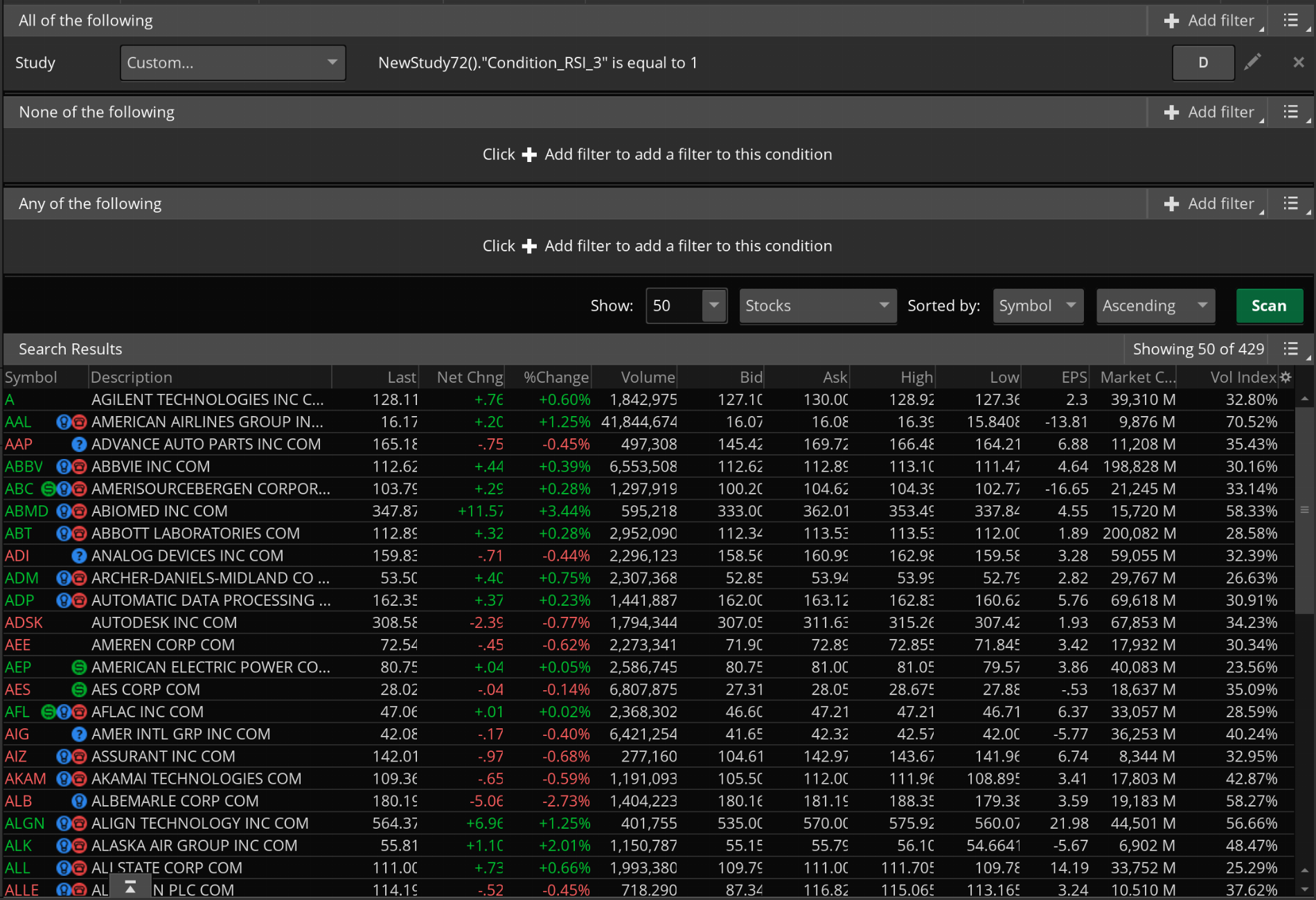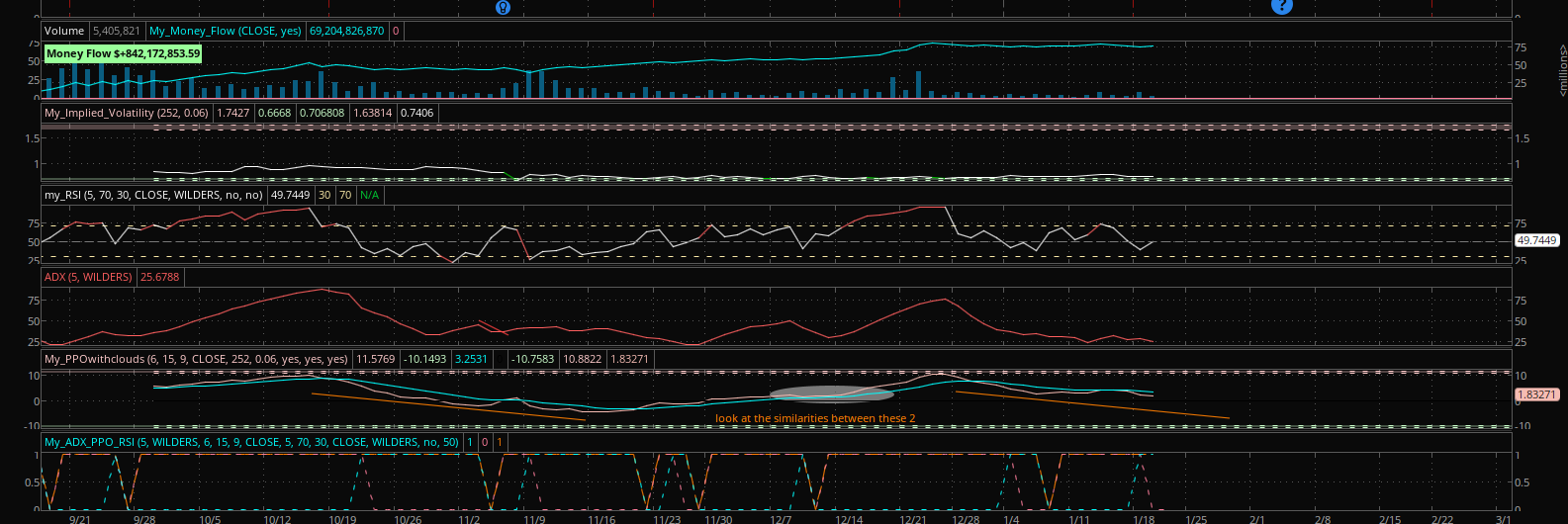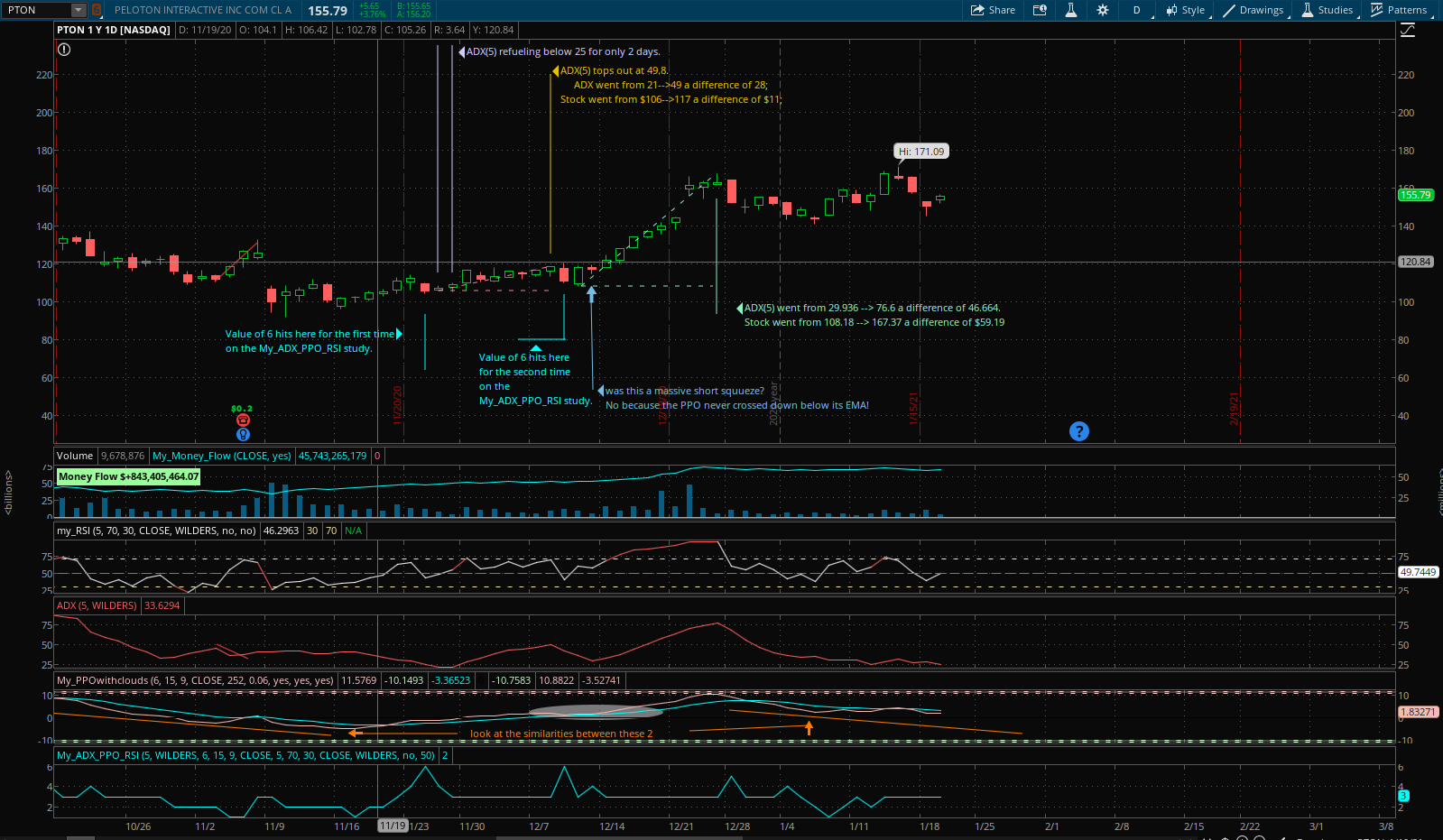# Trying to group multiple conditions into one scanner

#### mourningwood4521

##### Member
I want when:
& RSI < 50;
& RSI > 50;
&PPO > PPO;
&PPOEMA > PPOEMA;
& PPO > PPOEMA;
for my line/dataprofile to show a 1 when all these conditions are met and show a 0 if only one or any combination of these is not met.

When I string these together as individuals,
Code:
``````def rsi_1 = RSI < Trendline;
def rsi_2 = RSI > Trendline;
plot Condition_RSI_1 = RSI_1;
plot Condition_RSI_2 = RSI_2;``````

I have my 2 lines which follow their rules. when I input this:
Code:
``````def RSI_3 = if(rsi_1 + rsi_2) then 1 else 0 and if (RSI > Trendline or RSI < Trendline) then 0 else 1;
plot  Condition_RSI_3 = RSI_3;``````

It's not working and the line isn't going to zero when both of the conditions are not met.

Here is the script I have so far for the current one I'm having issues with. I'm including everything with the # and I've deleted a lot that wasn't working.

Code:
``````declare lower;
#-------------------------------------------------------------------------
#ppo and signal line
input fastPeriod   = 6;
input slowPeriod   = 15;
input signalPeriod = 9;
input price_1        = close;
def fastEma   = ExpAverage( price_1, fastPeriod );
def slowEma   = ExpAverage( price_1, slowPeriod );
def periodOK  = fastPeriod < slowPeriod;
AddLabel( !periodOK, "ERROR: fastPeriod MUST be less than slowPeriod" );
def ppo      = if periodOK then ((fastEma - slowEma) / slowEma) * 100 else 0;
def _signal   = ExpAverage( ppo, signalPeriod );
#----------------------
def PpoEma   = _signal;
def zeroLine = 0;
#----------------------------------------------------------------------------------
#RSI
input length = 5;
input over_Bought = 70;
input over_Sold = 30;
input price = close;
input averageType = AverageType.WILDERS;
input showBreakoutSignals = no;
input trendline =  50;
def NetChgAvg = MovingAverage(averageType, price - price, length);
def TotChgAvg = MovingAverage(averageType, AbsValue(price - price), length);
def ChgRatio = if TotChgAvg != 0 then NetChgAvg / TotChgAvg else 0;
def RSI = 50 * (ChgRatio + 1);
def OverSold = over_Sold;
def OverBought = over_Bought;
#def UpSignal = if RSI crosses above OverSold then OverSold else Double.NaN;
#def DownSignal = if RSI crosses below OverBought then OverBought else Double.NaN;
#----------------------------------------------------------------------------------
def rsi_1 = RSI < Trendline;
#def RSI_11 = RSI > Trendline;
def rsi_2 = RSI > Trendline;
#def RSI_21 =  RSI < Trendline;
#def RSI_3 = if(RSI < Trendline + RSI > Trendline) then 1 else 0 and if (RSI > Trendline or RSI < Trendline) then 0 else 1;
def RSI_3 = if(rsi_1 + rsi_2) then 1 else 0 and if (RSI > Trendline or RSI < Trendline) then 0 else 1;
def PPO_1 = ppo < ppo;
#def PPO_11 = ppo > ppo;
# and if(ppo > ppo) then 0 else 1 and if(PpoEma < PpoEma) then 0 else 1 and if(ppo > PpoEma) then 0 else 1 and if(ppo > ppo + PpoEma < PpoEma) then 0 else 1 and if(ppo > ppo + ppo > PpoEma) then 0 else 1 and if(PpoEma < PpoEma + ppo < PpoEma) then 0 else 1;
def PPO_2 = PpoEma > PpoEma;
#def PPO_22 = PpoEma < PpoEma;
def PPO_3 = ppo > PpoEma;
#def PPO_33 = ppo < PpoEma;
def PPO_4 = if (PPO_1 + PPO_2 + PPO_3) then 1 else 0;# and if (PPO_11 or PPO_22 or PPO_33) then 0 else 1;
#plot dataprofile_RSI= if (ADX_1 + RSI_1) then 1 else 0 and  if (ADX_1 or RSI_1) then 0 else 1;
##plot dataprofile_ADX_RSI = if(ADX_1 + RSI_3) then 1 else 0 and if(ADX_1 or RSI_3) then 0 else 1;
#Plot dataprofile_2 = RSI_3;
#plot dataprofile_3 = PPO_1 + PPO_2 + PPO_3;
#plot dataprofile_3 = PPO_4;

#plot dataprofile_4 = if(dataprofile + dataprofile_2 + dataprofile_3) then 1 else 0;
#plot condition_PPO_3 = if (ppo > PpoEma) then 1 else if (PpoEma > ppo) then 0 else 1;
#plot condition_PPO_2 = if (PpoEma > PpoEma) then 1 else 0 and if (PpoEma > PpoEma) then 0 else 1;
#plot condition_PPO_1 = if (ppo < ppo) then 1 else 0 and if (ppo > ppo) then 0 else 1;
#def  dataprofile_PPO_1 = condition_PPO_1;
#def  dataprofile_PPO_2 = condition_PPO_2;
#def  dataprofile_PPO_3 = condition_PPO_3;

#plot dataProfile_PPO   = if (dataprofile_PPO_1 + dataprofile_PPO_2 + dataprofile_PPO_3) then 1 else 0 and if (dataprofile_PPO_1 or dataprofile_PPO_2 or dataprofile_PPO_3) then 0 else double.NaN and if(dataprofile_PPO_1 + dataprofile_PPO_2) then 0 else double.NaN and if(dataprofile_PPO_1 + dataprofile_PPO_3) then 0 else double.NaN and if(dataprofile_PPO_1 + dataprofile_PPO_3) or dataprofile_PPO_1 or dataprofile_PPO_2 or dataprofile_PPO_3 then 0 else double.NaN;

#plot dataProfile_PPO   = dataprofile_PPO_1 + dataprofile_PPO_2 + dataprofile_PPO_3;
#plot dataprofile_
#---------
plot Condition_RSI_1 = RSI_1;
plot Condition_RSI_2 = RSI_2;
plot  Condition_RSI_3 = RSI_3;``````

Another study I made that is completely unrelated to this one is the following using what I believe is the same format because I tried to copy it.
And this study works perfectly fine on the chart and the version I converted to a scan inquiry is flawless.

Code:
``````declare lower;

input fastLength = 12;
input slowLength = 26;
input MACDLength = 9;
input averageTypeMACD = AverageType.EXPONENTIAL;
#input showBreakoutSignals = no;

def Diff = MACD(fastLength, slowLength, MACDLength, averageTypeMACD).Diff;
#plot UpSignal = if Diff crosses above 0 then 0 else Double.NaN;
#plot DownSignal = if Diff crosses below 0 then 0 else Double.NaN;

input over_bought = 80;
input over_sold = 20;
input KPeriod = 10;
input DPeriod = 10;
input priceH = high;
input priceL = low;
input priceC = close;
input averageTypeStoch = AverageType.SIMPLE;
#input showBreakoutSignals = {default "No", "On SlowK", "On SlowD", "On SlowK & SlowD"};

def SlowK = reference StochasticFull(over_bought, over_sold, KPeriod, DPeriod, priceH, priceL, priceC, 3, averageTypeStoch).FullK;

def SlowD = reference StochasticFull(over_bought, over_sold, KPeriod, DPeriod, priceH, priceL, priceC, 3, averageTypeStoch).FullD;

#plot DataProfile = (slowK < slowD) and (slowK > SlowD);

#plot DataProfile = (slowK < slowD) and (slowK > SlowD) and (lowest(SlowK, 3) < 50);

#plot DataProfile = (slowK < slowD) and (slowK > SlowD) and (lowest(SlowK, 3) < 70) and (Diff < Diff);

#plot chart = if((slowK < slowD) and (slowK > SlowD) and (lowest(SlowK, 3) < 50) and (Diff < Diff)) then 1 else 0;

def MACD = (Diff < Diff);

def Stoch_Slow = (SlowK < SlowD) and (SlowK > SlowD);

def Stoch_Slow_1 = (lowest(SlowK, 3) < SlowK);

def Stoch_Slow_2 = slowK > slowD;

#def Stoch_Slow_2 = highest(SlowK) > slowK;

#plot dataprofile = if(MACD + Stoch_Slow) then 1 else 0 and if(MACD or stoch_Slow)then 0 else 1;

plot dataprofile = if(MACD + Stoch_Slow + Stoch_Slow_1 + Stoch_Slow_2) then 1 else 0 and if(MACD or stoch_Slow or Stoch_Slow_1 or Stoch_Slow_2)then 0 else 1;``````

the # on the lines leading to the bottom is me showing my work on how I got there.

So, Im pretty confused why I can't combine the terms I want at the top on the script I am currently working with.

The scanner is working for me. What am I missing here?when I chart it, The orange line isn't doing what it should. The orange line should be at zero when both conditions are false or if only 1 condition is true. And the orange line should be at 1 when both conditions are true. currently RSI condition 1 is cyan and RSI Condition 2 is pink.

The line for both conditions is orange and should be at 1 when both conditions are true, and it's showing its at 1 for days a time when only 1 condition is true. that's what I don't get. I always make sure my graphs are squared away because the scan will get results no matter what and if the chart doesn't show what I want, the Scan won't be of any use to me.

I'm on my laptop and none of the 4 shareable Imgur links are working for me when I try to insert the links using the add image button.
edit got it to insert the photo.Last edited:
@mourningwood4521 I think you have a logic error in the following line of code... I have provided what I believe is the correct code...

Ruby:
``def RSI_3 = if(rsi_1 + rsi_2) then 1 else 0 and if (RSI > Trendline or RSI < Trendline) then 0 else 1;``

Ruby:
``def RSI_3 = if (rsi_1 + rsi_2) and (RSI > Trendline or RSI < Trendline) then 1 else 0;``

You cannot have multiple assignments in a single statement... Not sure if the logic is totally correct but I'm fairly certain that your line is incorrect...

Edited to add: I see what you're trying to do now... You're daisy-chaining logic conditionals which can be confusing... I'll dig deeper in a bit...

Last edited:
•mourningwood4521 and BenTen
@rad14733 RSI > Trendline is the opposite of RSI_1, where RSI_1 = RSI < Trendline. I will play around with the format you suggested and see if I can get the program to take the phrases. I started out with a format similar to what you suggested and it wouldn't take it, which is why I was using the second half.

Im thinking about reformatting it so every time one statement is true = 1, so if all 7 of my statements are true then I would have a graph line at 7, and say I have a bunch of days where 6 happen, then I can further refine my scan. I can also easily pick out other patterns that are occurring whereas if I just have a 0-1 oscillator, I wouldn't be able to pick out the other patterns.

Thank you for spending time looking into this for me.

So I decided to change it so I can see a value of 6 as having all of my parameters met. So here is a screenshot of what it looks like, and a current theory I'm working on. I haven't back tested this yet, so it could be nothing.Code:
``````#Hint: Shows a graph of 6 conditiojs when all 6 conditions are true you get a reading of 6.       File with the same name with Duplicate at the end contains all the knitty gritty on the PPO cloud data

declare lower;
#-------------------------------------------------------------------------
#ppo and signal line
input fastPeriod   = 6;
input slowPeriod   = 15;
input signalPeriod = 9;
input price_1        = close;
def fastEma   = ExpAverage( price_1, fastPeriod );
def slowEma   = ExpAverage( price_1, slowPeriod );
def periodOK  = fastPeriod < slowPeriod;
AddLabel( !periodOK, "ERROR: fastPeriod MUST be less than slowPeriod" );
def ppo      = if periodOK then ((fastEma - slowEma) / slowEma) * 100 else 0;
def _signal   = ExpAverage( ppo, signalPeriod );
#----------------------
def PpoEma   = _signal;
def zeroLine = 0;
#----------------------------------------------------------------------------------
#RSI
input length = 5;
input over_Bought = 70;
input over_Sold = 30;
input price = close;
input averageType = AverageType.WILDERS;
input showBreakoutSignals = no;
input trendline =  50;
def NetChgAvg = MovingAverage(averageType, price - price, length);
def TotChgAvg = MovingAverage(averageType, AbsValue(price - price), length);
def ChgRatio = if TotChgAvg != 0 then NetChgAvg / TotChgAvg else 0;
def RSI = 50 * (ChgRatio + 1);
def OverSold = over_Sold;
def OverBought = over_Bought;
#def UpSignal = if RSI crosses above OverSold then OverSold else Double.NaN;
#def DownSignal = if RSI crosses below OverBought then OverBought else Double.NaN;
#----------------------------------------------------------------------------------
def rsi_1 = if(RSI < Trendline) then 1 else 0;
def rsi_2 = if(RSI > Trendline) then 1 else 0;
def RSI_3 = if(rsi_1 + rsi_2) then 1 else 0;
def PPO_1 = if(ppo < ppo) then 1 else 0;
def PPO_2 = if(PpoEma > PpoEma) then 1 else 0;
def PPO_3 = if(ppo > PpoEma) then 1 else 0;

plot dataprofile = ADX_1 + RSI_1 + RSI_2 + PPO_1 + PPO_2 + PPO_3;``````

### Not the exact question you're looking for?

87k+ Posts
265 Online## The Market Trading Game Changer

Join 2,500+ subscribers inside the useThinkScript VIP Membership Club
• Exclusive indicators
• Proven strategies & setups
• Private Discord community
• Exclusive members-only content
• 1 full year of unlimited support

What is useThinkScript?

useThinkScript is the #1 community of stock market investors using indicators and other tools to power their trading strategies. Traders of all skill levels use our forums to learn about scripting and indicators, help each other, and discover new ways to gain an edge in the markets.

How do I get started?

We get it. Our forum can be intimidating, if not overwhelming. With thousands of topics, tens of thousands of posts, our community has created an incredibly deep knowledge base for stock traders. No one can ever exhaust every resource provided on our site.

If you are new, or just looking for guidance, here are some helpful links to get you started.

What are the benefits of VIP Membership?